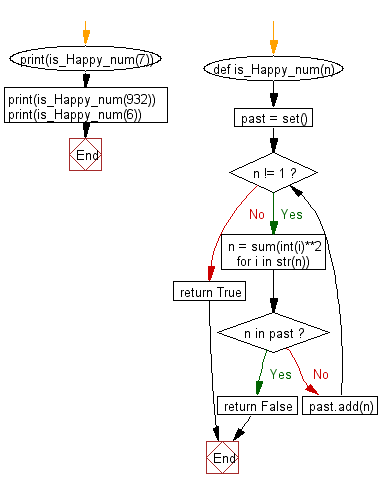﻿ Python: Check whether a number is "happy" or not - w3resource

# Python: Check whether a number is "happy" or not

## Python Basic - 1: Exercise-66 with Solution

From Wikipedia, the free encyclopaedia:
A happy number is defined by the following process:
Starting with any positive integer, replace the number by the sum of the squares of its digits, and repeat the process until the number equals 1 (where it will stay), or it loops endlessly in a cycle which does not include 1. Those numbers for which this process ends in 1 are happy numbers, while those that do not end in 1 are unhappy numbers.
Write a Python program to check whether a number is "happy" or not.

Sample Solution:

Python Code:

``````def is_Happy_num(n):
past = set()
while n != 1:
n = sum(int(i)**2 for i in str(n))
if n in past:
return False
return True
print(is_Happy_num(7))
print(is_Happy_num(932))
print(is_Happy_num(6))
``````

Sample Output:

```True
True
False
```

Pictorial Presentation:Flowchart:Python Code Editor:

Have another way to solve this solution? Contribute your code (and comments) through Disqus.

What is the difficulty level of this exercise?

Test your Programming skills with w3resource's quiz.

﻿